## Complexity classes of natural numbers (googology for ultrafinitists)

Ultrafinitists think common ways of defining extremely large numbers don’t actually refer to numbers that exist. For example, most ultrafinitists would maintain that a googolplex isn’t a number. But to a classical mathematician, while numbers like a googolplex are far larger than the numbers we deal with on a day-to-day basis like 10, both numbers have the same ontological status. In this post, I want to consider a compromise position, that any number we can define can be meaningfully reasoned about, but that a special status is afforded to the sorts of numbers that ultrafinitists can accept.

Specifically, define an “ultrafinite number” to be a natural number that it is physically possible to express in unary. This isn’t very precise, since there are all sorts of things that “physically possible to express in unary” could mean, but let’s just not worry about that too much. Also, many ultrafinitists would not insist that numbers must be expressible in such an austere language as unary, but I’m about to get to that.

Examples:is an ultrafinite number, because, whereis the successor function. 80,000 is also an ultrafinite number, but it is a large one, and it isn’t worth demonstrating its ultrafiniteness. A googol is not ultrafinite. The observable universe isn’t even big enough to contain a googol written in unary.

Now, define a “polynomially finite number” to be a natural number that it is physically possible to express using addition and multiplication. Binary and decimal are basically just concise ways of expressing certain sequences of addition and multiplication operations. For instance, “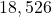” means. Conversely, if you multiply an-digit number with an-digit number, you get an at most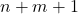-digit number, which is the same number of symbols it took write down “[the-digit number] times [the-digit number]” in the first place, so any number that can be written using addition and multiplication can be written in decimal. Thus, another way to define polynomially finite numbers is as the numbers that it is physically possible to express in binary or in decimal. I’ve been ignoring some small constant factors that might make these definitions not quite equivalent, but any plausible candidate for a counterexample would be an ambiguous edge case according to each definition anyway, so I’m not worried about that. Many ultrafinitists may see something more like polynomially finite number, rather than ultrafinite number, as a good description of what numbers exist.

Examples: A googol is polynomially finite, because a googol is 10000000000000000000000000000000000000000000000000000000000000000000000000000000000000000000000000000. A googolplex is not polynomially finite, because it would require a googol digits to express in decimal, which is physically impossible.

Define an “elementarily finite number” to be a number that it is physically possible to express using addition, multiplication, subtraction, exponentiation, and the integer division function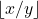. Elementarily finite is much broader than polynomially finite, so it might make sense to look at intermediate classes. Say a number is “exponentially finite” if it is physically possible to express using the above operations but without any nested exponentiation (e.g.is okay, butis not). More generally, we can say that a number is “-exponentially finite” if it can be expressed with exponentiation nested to depth at most, so a polynomially finite number is a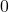-exponentially finite number, an exponentially finite number is a-exponentially finite number, and an elementarily finite number is a number that is-exponentially finite for some(or equivalently, for some ultrafinite).

Examples: a googolplex is exponentially finite, because it is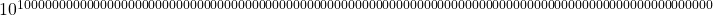. Thus a googolduplex, meaning, is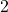-exponentially finite, but it is not exponentially finite. For examples of non-elementarily finite numbers, and numbers that are only-exponentially finite for fairly large, I’ll use up-arrow notation.just means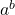,means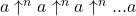, where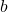is the number of copies of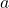, and using order of operations that starts on the right. So, which is certainly polynomially finite, and could also be ultrafinite depending on what is meant by “physically possible” (a human cannot possibly count that high, but a computer with a large enough hard drive can storein unary).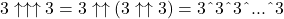, where there arethrees in that tower. Under the assumptions that implyis ultrafinite,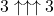is elementarily finite. Specifically, it is-exponentially finite, but I’m pretty sure it’s not-exponentially finite, or even-exponentially finite.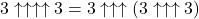, and is certainly not elementarily finite.

Interestingly, even though a googolplex is exponentially finite, there are numbers less than a googolplex that are not. There’s an easy nonconstructive proof of this: in order to be able to represent every number less than a googolplex in any encoding scheme at all, there has to be some number less than a googolplex that requires at least a googol decimal digits of information to express. But it is physically impossible to store a googol decimal digits of information. Therefore for any encoding scheme for numbers, there is some number less than a googolplex that cannot physically be expressed in it. This is why the definition of elementarily finite is significantly more complicated than the definition of polynomially finite; in the polynomial case, ifcan be expressed using addition and multiplication and, thencan also be expressed using addition and multiplication, so there’s no need for additional operations to construct smaller numbers, but in the elementary case, the operations of subtraction and integer division are useful for expressing more numbers, and are simpler than exponentiation. For example, these let us express the number that you get from reading off the last googol digits, or the first googol digits, of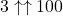, so these numbers are elementarily finite. However, it is exceptionally unlikely that the number you get from reading off the first googol decimal digits ofis elementarily finite. But for a difficult exercise, show that the number you get from reading off the last googol decimal digits ofis elementarily finite.

Why stop there instead of including more operations for getting smaller numbers, like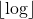, which I implicitly used when I told you that the number formed by the first googol digits ofis elementarily finite? We don’t have to. The functions that you can get by composition from addition, multiplication, exponentiation,, andcoincide with the functions that can be computed in iterated exponential time (meaning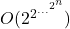time, for some height of that tower). So if you have any remotely close to efficient way to compute an operation, it can be expressed in terms of the operations I already specified.

We can go farther. Consider a programming language that has the basic arithmetic operations, if/else clauses, and loops, where the number of iterations of each loop must be fixed in advance. The programs that can be written in such a language are the primitive recursive functions. Say that a number is primitive recursively finite if it is physically possible to write a program (that does not take any input) in this language that outputs it. For each fixed, the binary function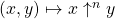is primitive recursive, sois primitive recursively finite. But the ternary functionis not primitive recursive, so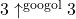is not primitive recursively finite.

The primitive recursively finite numbers can be put in a hierarchy of subclasses based on the depth of nested loops that are needed to express them. If the only arithmetic operation available is the successor function (from which other operations can be defined using loops), then the elementarily finite numbers are those that can be expressed with loops nested to depth at most 2. The-exponentially finite numbers should roughly correspond to the numbers that can be expressed with at mostloops at depth 2.

Next comes the provably computably finite numbers. Say that a number is provably computably finite if it is physically possible to write a program in a Turing-complete language that outputs the number (taking no input), together with a proof in Peano Arithmetic that the program halts. The famous Graham’s number is provably computably finite. Graham’s number is defined in terms of a function, defined recursively as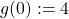and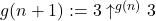. Graham’s number is. You could write a computer program to compute, and prove thatis total using Peano arithmetic. By replacing Peano arithmetic with other formal systems, you can get other variations on the notion of provably computably finite.

For an example of a number that is not provably computably finite, I’ll use the hydra game, which is described here. There is no proof in Peano arithmetic (that can physically be written down) that it is possible to win the hydra game starting from the complete binary tree of depth a googol. So the number of turns it takes to win the hydra game on the complete binary tree of depth a googol is not provably computably finite. If you start with a reasonably small hydra (say, with 100 nodes), you could write a program to search for the shortest winning strategy, and prove in Peano arithmetic that it succeeds in finding one, if you’re sufficiently clever and determined, and you use a computer to help you search for proofs. The proof you’d get out of this endeavor would be profoundly unenlightening, but the point is, the number of turns it takes to win the hydra game for a small hydra is provably computably finite (but not primitive recursively finite, except in certain trivial special cases).

Next we’ll drop the provability requirement, and say that a number is computably finite if it is physically possible to write a computer program that computes it from no input. Of course, in order to describe a computably finite number, you need the program you use to actually halt, so you’d need some argument that it does halt in order to establish that you’re describing a computably finite number. Thus this is arguably just a variation on provably computably finite, where Peano arithmetic is replaced by some unspecified strong theory encompassing the sort of reasoning that classical mathematicians tend to endorse. This is probably the point where even the most patient of ultrafinitists would roll their eyes in disgust, but oh well. Anyway, the number of steps that it takes to win the hydra game starting from the complete binary tree of depth a googol is a computably finite number, because there exists a shortest winning strategy, and you can write a computer program to exhaustively search for it.

The busy-beaver function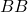is defined so thatis the longest any Turing machine withstates runs before halting (among those that do halt).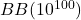is not computably finite, because Turing machines with a googol states cannot be explicitly described, and since the busy-beaver function is very fast-growing, no smaller Turing machine has comparable behavior. What about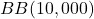? Turing machines with 10,000 states are not too big to describe explicitly, so it may be tempting to say thatis computably finite. But on the other hand, it is not possible to search through all Turing machines with 10,000 states and find the one that runs the longest before halting. No matter how hard you search and no matter how clever your heuristics for finding Turing machines that run for exceptionally long and then halt, it is vanishingly unlikely that you will find the 10,000-state Turing machine that runs longest before halting, let alone realize that you have found it. And the idea is to use classical reasoning for large numbers themselves, but constructive reasoning for descriptions of large numbers. So since it is pretty much impossible to actually write a program that outputs, it is not computably finite.

For a class that can handle busy-beaver numbers too, let’s turn to the arithmetically finite numbers. These are the numbers that are defined by arithmetical formulas. These form a natural hierarchy, where the-finite numbers are the numbers defined by arithmetical formulas with at mostunbounded quantifiers starting with, the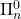-finite numbers are the numbers defined by arithmetical formulas with at mostunbounded quantifiers starting with, and the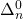-finite numbers are those that are both-finite and-finite. The-finite numbers are the same as the computably finite numbers.is-finite, because it is defined by “every Turing machine withstates that halts in at moststeps halts in at moststeps, and there is a Turing machine withstates that halts in exactlysteps.” Everything after the first quantifier in that formula is computable.is-finite, but no lower than that. To get a number that is not arithmetically finite, consider the functiongiven byis the largest number defined by an arithmetical formula withsymbols.is-finite, but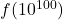is not arithmetically finite. I’ll stop there.